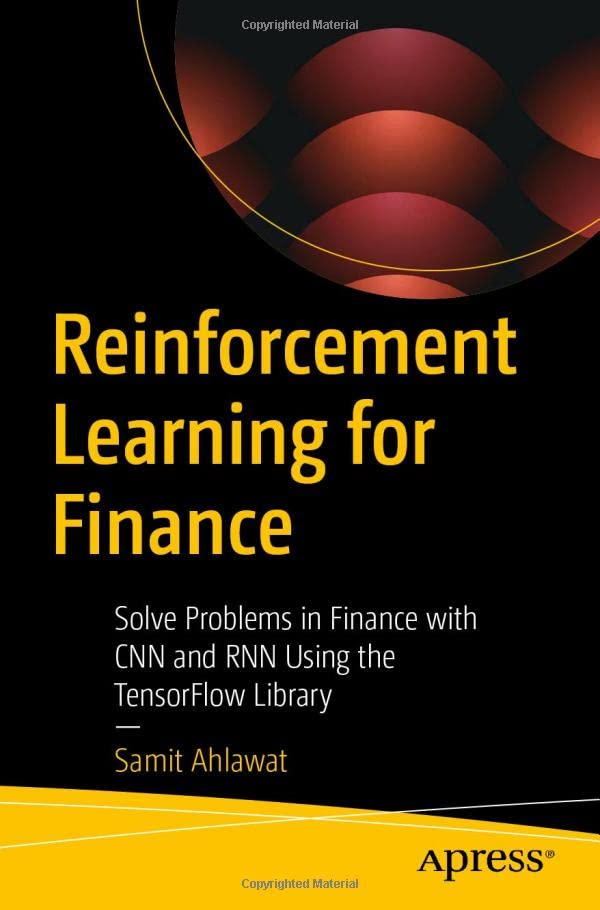# Reinforcement Learning for Finance: Solve Problems in Finance with CNN and RNN Using the TensorFlow Library

• Length: 438 pages
• Edition: 1
• Publisher:
• Publication Date: 2022-12-27
• ISBN-10: 1484288343
• ISBN-13: 9781484288344
Description

This book introduces reinforcement learning with mathematical theory and practical examples from quantitative finance using the TensorFlow library.

Reinforcement Learning for Finance begins by describing methods for training neural networks. Next, it discusses CNN and RNN – two kinds of neural networks used as deep learning networks in reinforcement learning. Further, the book dives into reinforcement learning theory, explaining the Markov decision process, value function, policy, and policy gradients, with their mathematical formulations and learning algorithms. It covers recent reinforcement learning algorithms from double deep-Q networks to twin-delayed deep deterministic policy gradients and generative adversarial networks with examples using the TensorFlow Python library. It also serves as a quick hands-on guide to TensorFlow programming, covering concepts ranging from variables and graphs to automatic differentiation, layers, models, and loss functions.

After completing this book, you will understand reinforcement learning with deep q and generative adversarial networks using the TensorFlow library.

What You Will Learn

• Understand the fundamentals of reinforcement learning
• Apply reinforcement learning programming techniques to solve quantitative-finance problems
• Gain insight into convolutional neural networks and recurrent neural networks
• Understand the Markov decision process

Who This Book Is ForData Scientists, Machine Learning engineers and Python programmers who want to apply reinforcement learning to solve problems.